Binary-Trees

 Question 1
If Tree-1 and Tree-2 are the trees indicated below :Which traversals of Tree-1 and Tree-2, respectively, will produce the same sequence?
 A Preorder, Postorder B Postorder, Inorder C Postorder, Preorder D Inorder, Preorder
Data-Structures       Binary-Trees       ISRO-2018
Question 1 Explanation:
→ Pre order traverses Root, Left, Right.
→ Post order traverses Left, Right, Root.
→ In-order traverses Left, Root, Right.
Tree-1: Post order: GJIHEFBDCA
Tree-2: In order: GJIHEFBDCA
 Question 2
Consider the following treeIf the post order traversal gives ab-cd*+ then the label of the nodes 1,2,3,… will be
 A +,-,*,a,b,c,d B a,-,b,+,c,*,d C a,b,c,d,-,*,+ D -,a,b,+,*,c,d
Data-Structures        Binary-Trees       ISRO-2017 May
Question 2 Explanation:
Step-1: Post order traversals yields from left,right and root.Step-2: The post order sequence is 4526731. The same we have to take it for above constraint.
Step-3: Then the sequence will be ab–cd*+ because 1= +, 2= -, 3= *, 4= a, 5=b, 6=c and 7=d.
 Question 3
A complete binary tree with the property that the value at each node is as least as large as the values at its children is known as
 A binary search tree B AVL tree C completely balanced tree D Heap
Data-Structures       Binary-trees       ISRO CS 2008
Question 3 Explanation:
In a Max. Binary Heap, the key value at each node is as least as large as the values at its children. Similarly in Min Binary Heap, the key at root must be minimum among all keys present in Binary Heap.
 Question 4
A full binary tree with n leaves contains:
 A n nodes B log2 n nodes C 2n-1 D 2n
Data-Structures       Binary-Trees       ISRO CS 2009
Question 4 Explanation:
A Binary Tree is full if every node has 0 or 2 children. So, in such case, the binary tree with n leaves contains a total of 2*n-1 nodes.Question 5
A complete binary tree with n non-leaf nodes contains
 A log2n nodes B n+1 nodes C 2n nodes D 2n+1 nodes
Data-Structures       Binary-Trees       ISRO-2016
Question 5 Explanation:
→ A binary tree where each non-leaf node has exactly two children. The number of leaves is n+1. Total number of nodes is 2n+1.
Note: if two leaves having the same parent are removed from the tree, the number of leaves and non-leaves will decrease by 1 because the parent will be a leaf. That means the difference between them is constant. And for a tree having just one node that difference is 1.
 Question 6
Which of the following number of nodes can form a full binary tree?
 A 8 B 15 C 14 D 13
Data-Structures       Binary-Trees       ISRO CS 2013
Question 6 Explanation:
A full binary tree is a tree in which every node other than the leaves has two children. In short, a full binary tree with N leaves contains 2N - 1 nodes.Question 7
How many different trees are there with four nodes A, B, C and D ?
 A 30 B 60 C 90 D None of the above
Data-Structures       Binary-Trees       ISRO CS 2014
Question 7 Explanation:
For a given nodes”n” , we can get nn-2 number of different trees
n=4. Here, n is number of nodes.
44-2=16.
Given options are wrong options.
Note: Correct answer is 16
 Question 8
A full binary tree with n non-leaf nodes contains
 A log​ 2​ n nodes B n+1 nodes C 2n nodes D 2n+1 nodes
Data-Structures       Binary-Trees       Nielit Scientist-C 2016 march
Question 8 Explanation:
● A full binary tree (sometimes proper binary tree or 2-tree) is a tree in which every node other than the leaves has two children
● In a binary tree - a tree where each non-leaf node has exactly two sons - number of leaves is n+1. Total number of nodes is 2n+1.
Full Binary Tree:In the above tree,non-leaf nodes are 7 so total nodes are 2x7+1=15
 Question 9
A balance factor in AVL tree is used to check
 A What rotation to make B if all childs nodes are at same level C when the last rotation occurred D if the tree is unbalanced
Data-Structures       Binary-Trees       Nielit Scientist-B CS 22-07-2017
Question 9 Explanation:
It is a self balancing tree with height difference at most 1. A balance factor in AVL tree is used to check what rotation to make.
 Question 10
traversing a binary tree first root and then left and right subtree called__ traversalt
 A postorder B preorder C inorder D none of these
Data-Structures       Binary-Trees       Nielit Scientist-B CS 2016 march
Question 10 Explanation:
In order:​ The left subtree is visited first, then the root and later the right subtree.
Pre order:​ The root node is visited first, then the left subtree and finally the right subtree.
Post order:​ First we traverse the left subtree, then the right subtree and finally the root node.
 Question 11
Which of the following need not be a binary tree?
 A Search tree B Heap C AVL tree D B tree
Data-Structures       Binary-Trees       Nielit Scientist-B CS 4-12-2016
Question 11 Explanation:
B trees need not be the binary tree. B trees may have more than 2 children. The order of B tree is maximum number of children a node can have.
 Question 12
The maximum number of nodes in a binary tree of level k, k>=1 is:
 A 2​ k​ +1 B 2​ k-1 C 2​ k​ -1 D 2​ k-1​ -1
Data-Structures       Binary-Trees       Nielit Scientist-B CS 4-12-2016
Question 12 Explanation:
The number of nodes is equal to 2​ k​ -1 where k≥1. So the minimum level is 1.
For exampleThe minimum number of nodes 2​ 2​ -1=3
 Question 13

Which of the following data structures is used in level order traversal of a binary tree?

 A Skip list B Stack C Array D Queue
Data-Structures       Binary-Trees       JT(IT) 2018 PART-B Computer Science
Question 13 Explanation:
Queue data structures is used in level order traversal of a binary tree.
Example:
Level order traversal of binary tree.
For each node, first the node is visited and then it’s child nodes are put in a FIFO queue.
printLevelorder(tree)
1) Create an empty queue q
2) temp_node = root /*start from root*/
3) Loop while temp_node is not NULL
a) print temp_node->data
b) Enqueue temp_node’s children (first left then right children) to q
c) Dequeue a node from q and assign it’s value to temp_node
Note:
Level order traversal of binary tree is time Complexity is O(n) where n is number of nodes in the binary tree.
 Question 14

In a full binary tree of height 10, the number of nodes with degree 0,1, and 2 will be ____,____ and ____ respectively.

Note: Consider height of a tree as the number of nodes in the longest path from root to any leaf node

 A 512, 0, 511 B 512, 1, 510 C 511, 0, 512 D 511, 1, 511
Data-Structures       Binary-Trees       JT(IT) 2018 PART-B Computer Science
Question 14 Explanation:
→ Height of the full binary tree 2h -1.
Full binary tree consists of either 0 or 2 childs . So one child to the node is not possible.
The number of nodes with the degree of 1 is 0.
The nodes with degree 0 are leaf nodes, 2h-1 = 29 = 512.
The nodes with degree 2 are internal nodes 2h-1 - 1 = 511.
 Question 15

Consider an AVL tree constructed by inserting the elements 18, 10, 5, 3, 27, 7, 35, 43, 32 one by one. Which of the following options give the number of comparisons to search the key value 45 in this AVL tree?

 A 5 B 4 C 3 D 2
Data-Structures       Binary-Trees       JT(IT) 2018 PART-B Computer Science
Question 15 Explanation: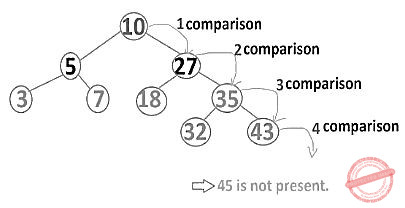Question 16
The height of a binary tree is the maximum number of edges in any root to leaf path. The maximum number of nodes in a binary tree of height h is
 A 2​ h B 2​ h-1​ -1 C 2​ h+1​ -1 D 2​ h+1
Data-Structures       Binary-Trees       Nielit Scientific Assistance IT 15-10-2017
Question 16 Explanation:
● The number of nodes n in a full binary tree, is at least n = 2 h + 1 and at most n = 2 h+1 − 1 , where h is the height of the tree. A tree consisting of only a root node has a height of 0.
● The number of leaf nodes l in a perfect binary tree, is l = ( n + 1 )/2 because the number of non-leaf (a.k.a. internal) nodes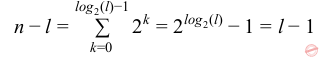● This means that a perfect binary tree with l leaves has n = 2 l − 1 nodes. ● In a balanced full binary tree, h = ⎡ log 2 (l)⎤ + 1 = ⎡ log 2 ((n + 1 )/2)⎤ + 1 = ⎡ log 2 (n + 1 )⎤
● In a perfect full binary tree, l = 2 h thus n = 2 h+1 − 1
● The maximum possible number of null links (i.e., absent children of the nodes) in a complete binary tree of n nodes is ( n + 1 ) , where only 1 node exists in bottom-most level to the far left.
●The number of internal nodes in a complete binary tree of n nodes is ⎣ n/2⎦ .
 Question 17
The number of possible binary trees with 4 nodes is
 A 12 B 13 C 14 D 15
Data-Structures       Binary-Trees       Nielit Scientific Assistance IT 15-10-2017
Question 17 Explanation:
There is one binary tree with one node. There are two differently shaped trees with two nodes. There are 14 different (shaped) binary trees with four nodes. These different trees are shown below.Question 18
A binary search tree contains the values- 1,2,3,4,5,6,7 and 8. The tree is traverses in preorder and the values are printed out. Which of the following sequences is a valid output?
 A 5 3 1 2 4 7 8 6 B 5 3 1 2 6 4 9 7 C 5 3 2 4 1 6 7 8 D 5 3 1 2 4 7 6 8
Data-Structures       Binary-Trees       Nielit Scientific Assistance CS 15-10-2017
Question 18 Explanation:
Preorder traversal yields (Root, left, right)
Option D:
Let draw binary search tree for the given sequence,After traversing through this tree we will get same sequence.
 Question 19
The figure below represents a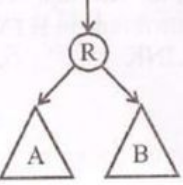A Binary tree B Recursive tree C Insert sort D Unitary tree
Data-Structures       Binary-Trees       KVS DEC-2013
Question 19 Explanation:
A binary tree is a tree data structure in which each node has at most two children, which are referred to as the left child and the right child
 Question 20
An important quantitative measure of the complexity of a binary tree is its__. It also provides a measure of the average depth of all nodes in the tree
 A Average path length B Median path length C Mode path length D Simple deviation path length
Data-Structures       Binary-Trees       KVS DEC-2013
Question 20 Explanation:
The number of decisions or, alternatively, the number of branches traversed, in reaching a node is called the path length or path to the node.
 Question 21
For the following binary tree, in operate the traversal yields the expressionA -+*/abdef B a+bd*-ef/ C abdef*/+- D a+b*d-e/f
Data-Structures       Binary-Trees       KVS DEC-2017
Question 21 Explanation:
Here, inoperate the traversal yields the expression means INORDER.
Inorder→ left,root and right.
a+b*d-c/f
 Question 22
A full binary tree with height h has _____ nodes.(Assume leaves at h=0)
 A 2h-1 B 2h+1 C 2h+1-1 D 2h+1+1
Data-Structures       Binary-Trees       KVS 30-12-2018 Part B
Question 22 Explanation:
→A full binary tree (sometimes proper binary tree or 2-tree) is a tree in which every node other than the leaves has two children.
→The height of the binary tree is the longest path from root node to any leaf node in the tree
 Question 23
Suppose you are given a binary tree with n nodes, such that each node has exactly either zero or two children. The maximum height of the tree will be
 A (n/2) -1 B (n/2) +1 C (n–1) / 2 D (n+1) / 2
Data-Structures       Binary-Trees       UGC NET CS 2016 July- paper-2
Question 23 Explanation:
→ They are given definition of full binary tree. The full binary tree each node has exactly either zero or two children.
→ The maximum height of the tree will be (n–1) / 2. It is standard property of full binary tree.
 Question 24
The inorder and preorder Traversal of binary Tree are dbeafcg and abdecfg respectively. The post-order Traversal is __________.
 A dbefacg B debfagc C dbefcga D debfgca
Data-Structures       Binary-Trees       UGC NET CS 2015 Jun- paper-2
Question 24 Explanation:
Inorder: Left root Right
Pre order: Root Left Right
Post order: Left Right Root
Inorder: d b e a f c gPre order: a b d e c f g
Post order: d e b f g c a
 Question 25
What item is at the root after the following sequence of insertions into an empty splay tree :
1, 11, 3, 10, 8, 4, 6, 5, 7, 9, 2, ?
 A 1 B 2 C 4 D 8
Data-Structures       Binary-Trees       UGC NET CS 2004 Dec-Paper-2
Question 25 Explanation:
A splay tree is a self-balancing binary search tree with the additional property that recently accessed elements are quick to access again. It performs basic operations such as insertion, look-up and removal in O(log n) amortized time. For many sequences of non-random operations, splay trees perform better than other search trees, even when the specific pattern of the sequence is unknown.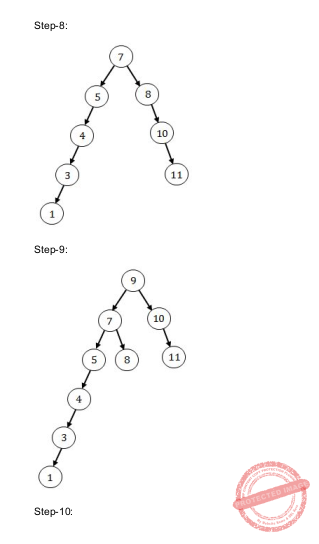Question 26
Which traversal techniques lists the nodes of a binary search tree in ascending order ?
 A post – order B in - order C pre - order D linear - order
Data-Structures       Binary-Trees       UGC NET CS 2005 Dec-Paper-2
Question 26 Explanation:
Inorder traversal technique in binary search tree will always produce ascending order.
Example:In this traversal method, the left subtree is visited first, then the root and later the right subtree. We should always remember that every node may represent a subtree itself.
 Question 27
In the balanced binary tree given below, how many nodes will become unbalanced when a node is inserted as a child of the node “g” ?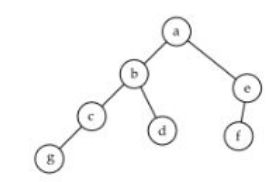A 1 B 3 C 7 D 8
Data-Structures       Binary-Trees       UGC NET CS 2005 june-paper-2
Question 27 Explanation:a, c, d are going to unbalance.
 Question 28
Binary search tree is an example of :
 A Divide and conquer technique B Greedy algorithm C Back tracking D Dynamic Programming
Data-Structures       Binary-Trees       UGC NET CS 2006 Dec-paper-2
Question 28 Explanation:
Binary search tree is an example of divide and conquer technique.
Procedure:
1. Divide: select lower or upper half
2. Conquer: search selected half
3. Combine: None
Recurrence relation​ : T(n)=T(n/2)+Θ(1)
Using masters theorem,
a=1, b=2, k=0,p=0
T(n)=Θ(logn)
 Question 29
In the balanced binary tree given below, how many nodes will become unbalanced when a node is inserted as a child of the node ​ ”g”.A 1 B 3 C 7 D 8
Data-Structures       Binary-Trees       UGC NET CS 2006 June-Paper-2
Question 29 Explanation:a, c, d are going to unbalance.
 Question 30
A full binary tree with n leaves contains
 A n nodes B log​ 2​ n nodes C 2n –1 nodes D 2​ n​ nodes
Data-Structures       Binary-Trees       UGC NET CS 2014 Dec-Paper-2
Question 30 Explanation:
A Binary Tree is full if every node has 0 or 2 children. So, in such case, the binary tree with n leaves contains a total of 2*n-1 nodes.There are 4 leaf nodes in the above tree.
So, total number of nodes are 2*4-1
=8-1
=7
 Question 31
The minimum number of nodes in a binary tree of depth d (root is at level 0) is
 A 2d – 1 B 2d + 1 – 1 C d + 1 D d
Data-Structures       Binary-Trees       UGC NET CS 2013 Sep-paper-2
Question 31 Explanation:
Binary tree is a tree where each node has at most 2 children nodes.
Properties:
→The number of nodes at depth d in a perfect binary tree = 2d
→A perfect binary tree of height h has 2h+1 -1 nodes
→Number of leaf nodes in a perfect binary tree of height h = 2h
→Number of internal nodes in a perfect binary tree of height h = 2h -1
→The minimum number of nodes in a binary tree of height h = h+1
→The maximum number of nodes in a binary tree of height h = 2h+1 -1
 Question 32
Consider the In-order and Post-order traversals of a tree as given below :
In-order : j e n k o p b f a c l g m d h I
Post-order : j n o p k e f b c l m g h i d a
The Pre-order traversal of the tree shall be
 A a b f e j k n o p c d g l m h i B a b c d e f j k n o p g l m h i C a b e j k n o p f c d g l m h i D j e n o p k f b c l m g h i d a E None of the above
Data-Structures       Binary-Trees       UGC NET CS 2013 Sep-paper-2
Question 32 Explanation:
Step-1: Post order traversal is Left, Right and Root according to post order we are dividing into in-order list.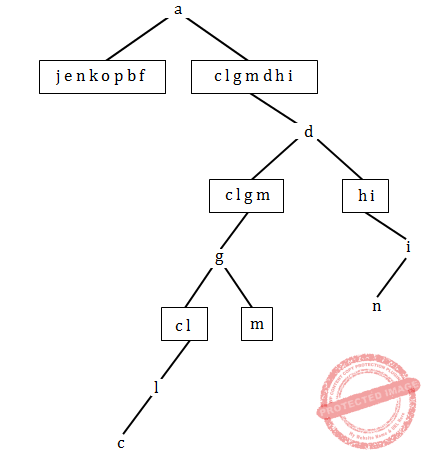Step-2:Step-3: Final tree isStep-4: Preorder traversal is Root,left and right
preorder= a b e j k n p o f d g l c m i h
Note: Given options are wrong. Excluded for evaluation.
 Question 33
The worst case time complexity of AVL tree is better in comparison to binary search tree for
 A Search and Insert Operations B Search and Delete Operations C Insert and Delete Operations D Search, Insert and Delete Operations
Data-Structures       Binary-Trees       UGC NET CS 2012 Dec-Paper-2
Question 33 Explanation:
AVL tree is a self balancing tree with height difference at most 1. A balance factor in AVL tree is used to check what rotation to make.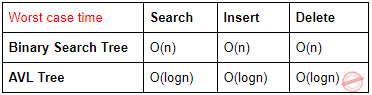Question 34
In which tree, for every node the height of its left subtree and right subtree differ almost by one ?
 A Binary search tree B AVL tree C Threaded Binary Tree D Complete Binary Tree
Data-Structures       Binary-Trees       UGC NET CS 2012 Dec-Paper-2
Question 34 Explanation:
→ AVL tree is a self balancing tree with height difference at most 1. A balance factor in AVL tree is used to check what rotation to make.
→ ALV tree
 Question 35
Consider the tree given below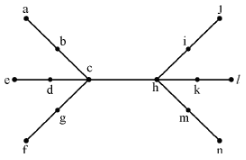Using the property of eccentricity of a vertex, find every vertex that is the centre of the given tree.
 A d & h B c & k C g, b, c, h, i, m D c & h
Data-Structures       Binary-Trees       UGC NET CS 2012 Dec-Paper-2
Question 35 Explanation: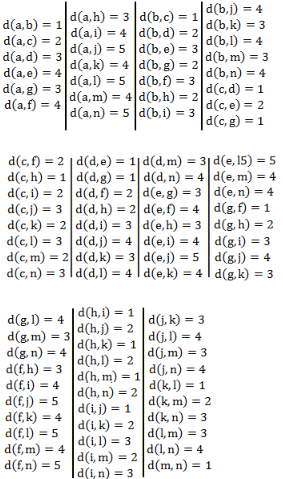Eccentricity of a vertex u, is
E(u) = maxu∈v(G) d(u, v)
i.e., the maximum distance of a node to other nodes is the eccentricity of that node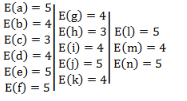Radius: The minimum eccentricity from all the vertices is considered as the radius of the Graph G denoted by r(G).
Here radius of the graph, r(G)=3
Center:- If the eccentricity of a graph is equal to its radius, then it is known as the central point of the graph.
And in above graph vertex "h" and " c" have eccentricity equal to the radius of the graph. So vertex h and c are the center of graph.
Hence correct answer is option (D).
 Question 36
The post order traversal of a binary tree is DEBFCA. Find out the preorder traversal.
 A ABFCDE B ADBFEC C ABDECF D None of the above
Data-Structures       Binary-Trees       UGC NET CS 2012 June-Paper2
Question 36 Explanation:
Post order traversal tree is look like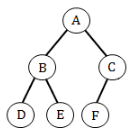Postorder: DEBFCA
Inorder: DBEAFC
Preorder: ABDECF
 Question 37
A binary search tree is a binary tree :
 A All items in the left subtree are less than root B All items in the right subtree are greater than or equal to the root C Each subtree is itself a binary search tree D All of the above
Data-Structures       Binary-Trees       UGC NET CS 2012 June-Paper2
Question 37 Explanation:
Binary search tree properties: 1. All items in the left subtree are less than root
2. All items in the right subtree are greater than or equal to the root
3. Each subtree is itself a binary search tree
 Question 38
To represent hierarchical relationship between elements, which data structure is suitable ?
 A Dequeue B Priority C Tree D All of the above
Data-Structures       Binary-Trees       UGC NET CS 2012 June-Paper2
Question 38 Explanation:
Double-Ended Queue is a data structure in which insertion and deletion of data takes place from different ends. The insertion of data takes place from REAR end and deletion of data takes place from FRONT end. It follows FIFO( First In First Out) order for implementation.Priority Queue is like a regular queue or stack data structure, but here each element has a "priority" associated with it. In a priority queue, an element with high priority is served before an element with low priority.
Tree: A tree is a data structure containing a collection of nodes. Here a node can have any number of children. A node with no child is called as leaf node and a node with 1 or more child is called parent node. Each parent node have a pointer to its children.Question 39
The post order traversal of a binary tree is DEBFCA. Find out the preorder traversal.
 A ABFCDE B ADBFEC C ABDECF D ABDCEF
Data-Structures       Binary-Trees       UGC NET CS 2011 Dec-Paper-2
Question 39 Explanation:
Post order traversal tree is look likePostorder: DEBFCA
Inorder: DBEAFC
Preorder: ABDECF
 Question 40
The number of nodes in a complete binary tree of height h (with roots at level 0) is equal to
 A 20 + 21 + ….. 2h B 20 + 21 + ….. 2h – 1 C 20 + 21 + ….. 2h + 1 D 21 + ….. 2h + 1
Data-Structures       Binary-Trees       UGC NET CS 2011 Dec-Paper-2
Question 40 Explanation:
The number of nodes in a complete binary tree of height h (with roots at level 0) is equal to 20 + 21 + ….. 2h. because every phase we are getting 2h.
Suppose, in level-3, it have 23=8 nodes.
 Question 41
The number of different trees with 8 nodes is
 A 256 B 255 C 248 D None of these
Data-Structures       Binary-Trees       UGC NET CS 2011 June-Paper-2
Question 41 Explanation:
Given data
-- nodes=8
-- Total number of trees=?
Possibility-1: To find total number of trees using nn-2.
= 88-2
= 262144 trees are possible.
Possibility-2: According to given problem, they are using binary tree based on below formula
= 2n -n

= 28 -8
= 248
Note: They are not given proper input.
 Question 42
Given a binary tree whose inorder and preorder traversal are given by
In order : E I C F B G D J H K
Preorder : B C E I F D G H J K
The post order traversal of the above binary tree is
 A I E F C G J K H D B B I E F C J G K H D B C I E F C G K J H D B D I E F C G J K D B H
Data-Structures       Binary-Trees       UGC NET CS 2011 June-Paper-2
Question 42 Explanation:Question 43
A binary tree with 27 nodes has _______ null branches.
 A 54 B 27 C 26 D None of the above
Data-Structures       Binary-Trees       UGC NET CS 2010 Dec-Paper-2
 Question 44
In a complete binary tree of n nodes, how far are the two most distant nodes? Assume each edge in the path counts as !
 A About log2n B About 2 log2n C About n log2n D About 2n
Data-Structures       Binary-Trees       UGC NET CS 2010 June-Paper-2
Question 44 Explanation:
According to binary two most distinct nodes are in
level-1: 1 and 2
level-2: 3 and 6.
[Note: Assume that root starts from level-0]The height of the binary tree is O(log2n). As per the given constraint, we have to calculate individually of left farthest node and right farthest node height.
=log2n*log2n
=2*log2n or (log2n)2
 Question 45
In a full binary tree of height k, there are __________ internal nodes.
 A 2k-1 B 2k-1 C 2k D 2k+1
Data-Structures       Binary-Trees       UGC NET CS 2009-June-Paper-2
Question 45 Explanation:
→ In a full binary tree of height k, there are 2k-1 internal nodes.
→ A Binary Tree is full if every node has 0 or 2 children.
→ In a Full Binary, number of leaf nodes is number of internal nodes plus 1
L = I + 1
Where,
L = Number of leaf nodes,
I = Number of internal nodes.
 Question 46
A binary tree is said to have heap property if the elements along any path :
 A from leaf to root are non–increasing B from leaf to root are non–decreasing C from root to leaf are non–decreasing D from root to leaf are non–increasing
Data-Structures       Binary-Trees       UGC NET CS 2009-June-Paper-2
Question 46 Explanation:
A heap is a complete binary tree. A binary tree is said to have heap property if the elements along any path from root to leaf are non–increasing
 Question 47
Which of the following can be the sequence of nodes examined in a binary search tree while searching for key 98 ?
 A 100,50,75, 60, 98 B 100, 120, 90, 95, 98 C 200,70,100, 95, 98 D 75, 150, 90, 80, 98
Data-Structures       Binary-Trees       UGC NET CS 2008-june-Paper-2
Question 47 Explanation:
Binary search tree will follow some rules
1. Left subtree is smaller than his root node
2. Right subtree is greater than or equal to his root
Based on above rules, the search operation will perform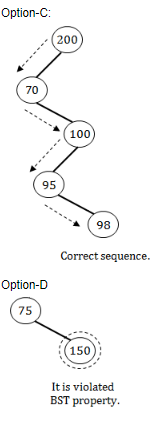Question 48
The height of a binary tree with ‘n’ nodes, in the worst case is :
 A O(log n) B O(n) C Ω(n log n) D Ω (n2)
Data-Structures       Binary-Trees       UGC NET CS 2007-Dec-Paper-2
Question 48 Explanation:
The height of a binary tree with ‘n’ nodes, in the worst case is O(n). Sometimes, it will get left skewed/ right skewed based on given input.Question 49
The maximum number of nodes in a binary tree of depth 10 :
 A 1024 B 210 - 1 C 1000 D None of the above
Data-Structures       Binary-Trees       UGC NET CS 2007 June-Paper-2
Question 49 Explanation:
→ The depth of binary tree starts from 0 but not 1.
→ To find maximum number of nodes in a binary tree of depth using formula is
= 2h+1 -1
= 211 -1
= 2048-1
= 2047Question 50
Consider a full binary tree with n internal nodes, internal path length i, and external path length e. The internal path length of a full binary tree is the sum, taken over all nodes of the tree, of the depth of each node. Similarly, the external path length is the sum, taken over all leaves of the tree, of the depth of each leaf. Which of the following is correct for the full binary tree?
 A e = i+n B e = i+2n C e = 2i+n D e = 2n+i
Data-Structures       Binary-Trees       UGC NET CS 2017 Nov- paper-3
Question 50 Explanation:
→ A node's path length is the number of links (or branches) required to get back to the root.
→ The root has path length zero and the maximum path length in a tree is called the tree's height.
→ The sum of the path lengths of a tree's internal nodes is called the internal path length and the sum of the path lengths of a tree's external nodes is called the external path length.
External Path Length: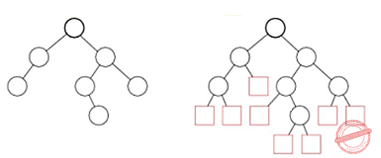The sum over all external (square) nodes of the lengths of the paths from the root of an extended binary tree to each node. For example, in the tree above, the external path length is 25 (Knuth 1997, pp. 399-400). The internal and external path lengths are related by
E = I + 2n,
where n is the number of internal nodes.
 Question 51
The number of different binary trees with 6 nodes is ______.
 A 6 B 42 C 132 D 256
Data-Structures       Binary-Trees       UGC NET CS 2016 July- paper-3
Question 51 Explanation:
Using catalan formula to find the total number of binary trees is (2n)! / (n! * (n+1)!)

Here, n=6

= (2*6)! / (6! * (6+1)!)

= (12)! / (6! * (7)!)

= 479001600 / (720*5040)

= 132

 Question 52
Suppose that we have numbers between 1 and 1,000 in a binary search tree and want to search for the number 364. Which of the following sequences could not be the sequence of nodes examined ?
 A 925, 221, 912, 245, 899, 259, 363, 364 B 3, 400, 388, 220, 267, 383, 382, 279, 364 C 926, 203, 912, 241, 913, 246, 364 D 3, 253, 402, 399, 331, 345, 398, 364
Data-Structures       Binary-Trees       UGC NET CS 2016 July- paper-3
Question 52 Explanation:There are 52 questions to complete.
PHP Code Snippets Powered By : XYZScripts.com